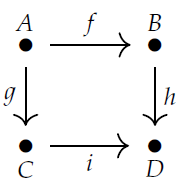Julio - I guess you're happy now, but a "diagram of shape \$$\mathcal{A}\$$ in the category \$$\mathcal{B}\$$" is just a functor \$$F : \mathcal{A} \to \mathcal{B}\$$. (I'm deliberately using completely different letters, because you should never get attached to particular letters.)

So, for example, there's a category \$$\mathcal{A}\$$ called the **square**:and we can look at a diagram of this shape in any category \$$\mathcal{B}\$$. Very roughly speaking, it's like a picture of shape \$$\mathcal{A}\$$ drawn on the canvas \$$\mathcal{B}\$$. This is a very important and beautiful idea.